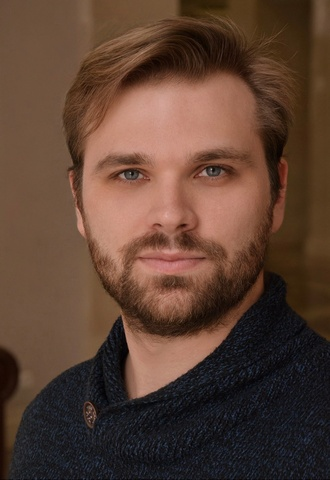# Andrey Selutin

### ScheduleSpecial prize of the Irina Arkhipova Foundation at the 1st International Festival Competition for Young Singers вЂњMusic of FriendshipвЂќ (Voronezh, Russia, 2016)В

Andrey SelutinВ is a graduate in voice of the MichaЕ‚ OgiЕ„ski Molodechno State College of Music (2011) and the Belarusian State Academy of Music (class of the Honoured Artist of the Republic of Belarus Oleg Melnikov, 2018).

In 2016, he joined the young artist programme of the Bolshoi Theatre of Belarus, followed by his promotion to soloist of the opera company in 2018.

### REPERTOIRE

BasilioВ В В В В В В В В В В В В В В В В В В В В В В В В В В В В В В В В В В В В В В В В В В В В В В В В В В В В В В В В В  Il barbiere di Siviglia

The Robber ChiefВ В В В В В В В  The Story of Kai and Gerda (The Snow Queen) by Sergei Banevich

DulcamaraВ В В В В В В В В В В В В В В В В В В В В В В В В В  В В В В В В В В В В В В В В В В В В В В В В В В В В В L'elisir d'amore

AbimГ©lechВ  В  В  В  В  В  В  В  В  В  В  В  В  В  В  В  В  В  В  В  В  В  В  В  В  В  В В Samson et Dalila
Ales VaronaВ  В  В  В  В  В  В  В  В  В  В  В  В  В  В  В  В  В  В  В  В  В  В  В  В  В  King Stakh's Wild Hunt by Vladimir Soltan
EscamilloВ В В В В В В В В В В В В В В В В В В В В В В В В В В В В В В В В В В В В В В В В В В В В В В В В В В В В В В  Carmen

The CatВ  В  В  В  В  В  В  В  В  В  В  В  В  В  В  В  В  В  В  В  В  В  В  В  В  В  В  В  В  В Pinocchio by Gloria Bruni
MГ©phistophГ©lГЁs В В В В В В В В В В В В В В В В В В В В В В В В В В В В В В В В В В В В В В В В В В В В В Faust

The SacristanВ  В  В  В  В  В  В  В  В  В  В  В  В  В  В  В  В  В  В  В  В  В  В  В  В В Tosca

Don PasqualeВ В В В В В В В В В В В В В В В В В В В В В В В В В В В В В В В В В В В В В В В В В В В В В В В В В  Don Pasquale

The Baron В В В В В В В В В В В В В В В В В В В В В В В В В В В В В В В В В В В В В В В В В В В В В В В В В В В В В В В La traviata

CollineВ В В В В В В В В В В В В В В В В В В В В В В В В В В В В В В В В В В В В В В В В В В В В В В В В В В В  В В В В В В В В В La bohГЁme

The Speaker, the Armoured Man, the Priest В В В Die ZauberflГ¶te

The Company CommanderВ В В В В В В В В В В В В В В В В В В В В В В В В В В В В  Eugene Onegin

Wise MenВ В В В В В В В В В В В В В В В В В В В В В В В В В В В В В В В В В В В В В В В В В В В В В В В В В В В В В В В  Turandot

SoldierВ В В В В В В В В В В В В В В В В В В В В В В В В В В В В В В В В В В В В В В В В В В В В В В В В В В В В В В В В В В В В  Salome

### CONCERT TOUR

In October 2022, he performed the role of Ales Varona in King StakhвЂ™s Wild Hunt, a guest performance of the Bolshoi Theatre of Belarus on the Historic Stage of the Bolshoi Theatre of Russia.

В В

### Partners